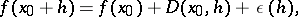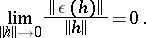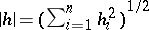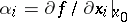# Fréchet differential

(diff) ← Older revision | Latest revision (diff) | Newer revision → (diff)

at a pointof a mappingof a normed spaceinto a normed spaceThe mappingwhich is linear and continuous fromintoand has the property that(1)

whereIf a mappingadmits an expansion (1) at a point, then it is said to be Fréchet differentiable, and the actual operatoris called the Fréchet derivative.

For a functionin a finite number of variables, the Fréchet differential is the linear functionthat has the property that(2)

whereor any other equivalent norm in. Hereare the partial derivatives ofat.

Definition (2), which is now commonplace, apparently first appeared in an explicit form in the lectures of K. Weierstrass (1861, see ). At the end of the 19th century this definition gradually came into the textbooks (see ,  and others). But at the time when M. Fréchet began to develop infinite-dimensional analysis, the now classical definition of the differential was so far from commonplace that even Fréchet himself supposed that his definition of the differential in an infinite-dimensional space was a new concept in the finite-dimensional case too. Nowadays the term is only used in relation to infinite-dimensional mappings. See Gâteaux differential; Differential.

How to Cite This Entry:
Fréchet differential. Encyclopedia of Mathematics. URL: http://encyclopediaofmath.org/index.php?title=Fr%C3%A9chet_differential&oldid=14772
This article was adapted from an original article by V.M. Tikhomirov (originator), which appeared in Encyclopedia of Mathematics - ISBN 1402006098. See original article